login  home  contents  what's new  discussion  bug reports help  links  subscribe  changes  refresh  edit

On January 19, 2006 5:53 AM Fabio Stumbo wrote:

## if-then-else: what is wrong?

I am having some problem with the if-then-else syntax in the .input files.

Problem 1: I refer to the axiom book which ships with the program (version 2005/09). At page 204 it says that the usual rules for piling are suspended in conditional expressions and it gives 5 different ways which should be equivalent, namely::

fricas
i := 2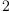(1)
Type: PositiveInteger?
fricas
if i>0 then output("positive") else output("nonpositive")
positive
Type: Void
fricas
if i > 0 then output("positive")
else output("nonpositive")
positive
Type: Void
fricas
if true then
if i > 0 then output("positive")
else output("nonpositive")
positive
Type: Void
fricas
if true then
if i > 0
then output("positive")
else output("nonpositive")
positive
Type: Void
fricas
if i > 0
then output("positive")
else output("nonpositive")
Line  15:
Line  16: if i > 0
Line  17:     then output("positive")
....A
Error  A: (from A and on) Ignored from here
Error  A: Improper syntax.
Line  18:     else output("nonpositive")
.............................A
Error  A: (up to A) to here.
Error  A: syntax error at top level
Error  A: Possibly missing a then
5 error(s) parsing

Well, I tried all of them, creating each time a blank r.input file with inside only:

fricas
i := 2(2)
Type: PositiveInteger?

followed by one of the "if...".

The result is that the first two are ok, where as the other three give some syntax error (different each time).

fricas
for i in 2..2 repeat
if i>0 then output("positive") else output("nonpositive")
positive
Type: Void

fricas
for i in 2..2 repeat
if i > 0 then output("positive")
else output("nonpositive")
positive
Type: Void

fricas
for i in 2..2 repeat
if i > 0 then output("positive")
else output("nonpositive")
positive
Type: Void

fricas
for i in 2..2 repeat
if i > 0
then output("positive")
else output("nonpositive")
positive
Type: Void

The ones above works OK. The following one looks like a bug...

fricas
for i in 2..2 repeat
if i > 0
then output("positive")
else output("nonpositive")
Line   1: for i in 2..2 repeat
Line   2:   if i > 0
..A
Error  A: (from A and on) Ignored from here
Line   3:     then output("positive")
....A
Error  A: Improper syntax.
Error  A: (from A and on) Ignored from here
Line   4:     else output("nonpositive")
.............................A
Error  A: (up to A) to here.
Error  A: Possibly missing a then
Error  A: (up to A) to here.
6 error(s) parsing

fricas
for i in 2..2 repeat
if i > 0 then
output(i)
output("positive")
else
output(i)
output("nonpositive")
2
positive
Type: Void

fricas
i:=1.5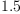(3)
Type: Float
fricas
a :=
if i > 0 then
j := sin(i * pi())
exp(j + 1/j)
else
j := cos(i * 0.5 * pi())
log(abs(j)**5 + 1)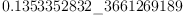(4)
Type: Float

Problem 2: again with if then else, in a complex function I noticed a wrong result (the function itself was ok... ;-) Simplifying, you can produce the same error with something like:

fricas
test: (INT,INT) -> List(INT)
Type: Void
fricas
test(a,b) ==
x := 0; y := 0
if (a rem b = 0) and b < 0 then
x := 1
y := 1
[x,y]
Type: Void

Then,

fricas
4 rem -2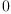(5)
Type: NonNegativeInteger?
fricas
test(4,-2)
fricas
Compiling function test with type (Integer, Integer) -> List(Integer
)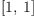(6)
Type: List(Integer)

returns correctly '[1,1]?', but

fricas
4 rem -3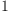(7)
Type: PositiveInteger?
fricas
test(4,-3)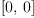(8)
Type: List(Integer)

or

fricas
4 rem 2(9)
Type: NonNegativeInteger?
fricas
test(4,2)(10)
Type: List(Integer)

returns wrongly '[0,1]?'. Actually the result is '[0, 0]?' which is correct.

The same happens if I write

fricas
test: (INT,INT) -> List(INT)
Compiled code for test has been cleared.
Type: Void
fricas
test(a,b) ==
x := 0; y := 0
if (a rem b = 0) and b < 0 then  x := 1 ; y := 1
[x,y]
1 old definition(s) deleted for function or rule test
Type: Void

Why?

Another small question. Is there a way to obtain on line the syntax of a command in a more extensive way? I mean, for example, that writing

fricas
)di op extendedEuclidean
There are 2 exposed functions called extendedEuclidean :
 (D,D,D) -> Union(Record(coef1: D,coef2: D),"failed") from D
if D has EUCDOM
 (D,D) -> Record(coef1: D,coef2: D,generator: D) from D if D has
EUCDOM

which is not too useful. Moreover, how can I just ask about, say, the second function extendedEuclidean?

If I wanted to ask about the syntax of if-then-else, I would like to write something like:

  )? if
or
)ap if


but nothing gives clues on how to use it.

Thank you for all your help

 Subject:   Be Bold !! ( 14 subscribers )